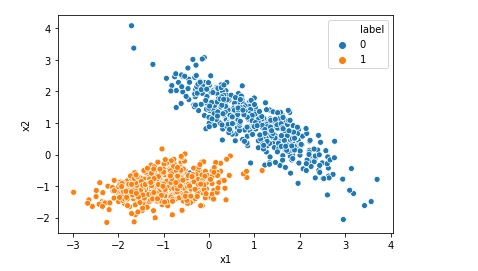Visualize or Print Random Forest Algorithm Model

As a machine learning engineer you may have created the Random Forest Algorithm Model, but have you ever tried to visualize it. If not , this is the post for you. In this post we will learn how to Visualize or Print Random Forest Algorithm Model in Jupyter notebook. https://www.youtube.com/watch?v=6uPavHNuatA&t=1s Top 10 Free Books for … Continue reading Visualize or Print Random Forest Algorithm ModelRandom Forest Classification in Python in 10 Lines

Random Forest algorithm is like an ensemble algorithm made of Decision Trees, which comprises more than one decision tree to create a model. It creates more than one tree like conditional control statements to create its model hence it is named as Random Forest. Random Forest machine learning algorithm can be used to solve both … Continue reading Random Forest Classification in Python in 10 LinesDecision Tree Classification in Python in 10 lines

Decision tree machine learning algorithm can be used to solve not only regression but also classification problems. This algorithm creates a tree like conditional control statements to create its model hence it is named as decision tree. In this post we will be implementing a simple decision tree classification model using python and sklearn. First … Continue reading Decision Tree Classification in Python in 10 linesPrint ROC AUC Receiver Operating Characteristic Area Under Curve

The receiver operating characteristic area under curve is a way to measure the performance of a classification model, may be created using algorithms like Logistic Regression. ROC-AUC is basically a graph where we plot true positive rate on y-axis and false positive rate on x-axis. If a model is good the AUC will be close to 1. Area … Continue reading Print ROC AUC Receiver Operating Characteristic Area Under CurveScaling Data Range using Min Max Scaler

Suppose you have a dataset that has float values and all values in the range 0 to 1. You want to change all values to integer with a range between 10 to 20. In this post we will learn how to do this using MinMaxScaler Data before scaling Now let us scale the data as … Continue reading Scaling Data Range using Min Max ScalerCreating Synthetic Data for Logistic Regression

Many a times we want to implement Logistic Regression on certain data but we do not find that kind of data online. In that case we can generate a synthetic data for our problem. In this post we will see how to generate a typical synthetic data for a simple Logistic Regression. Import the required … Continue reading Creating Synthetic Data for Logistic RegressionLinear Regression Synthetic Data using Make Regression

Though we have many datasets available on internet for implementing Linear Regression , many a times we may require to create a our own synthetic data. Scikit-Learn has a class called make regression , we can use this class to generate synthetic data for linear regression. from sklearn.datasets import make_regression # generate regression dataset x, … Continue reading Linear Regression Synthetic Data using Make RegressionImplementing Logistic Regression in 10 lines in Python

Logistic Regression is one of the most popular Machine Learning algorithm used for the classification problems. It should be noted that though there is a regression word in the name of the algorithm Logistic Regression, it is used for classification problems. A use case of Logistic regression could be, based on the symptoms for a disease that a patient has Logistic … Continue reading Implementing Logistic Regression in 10 lines in Python